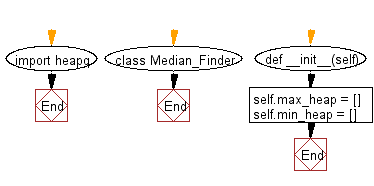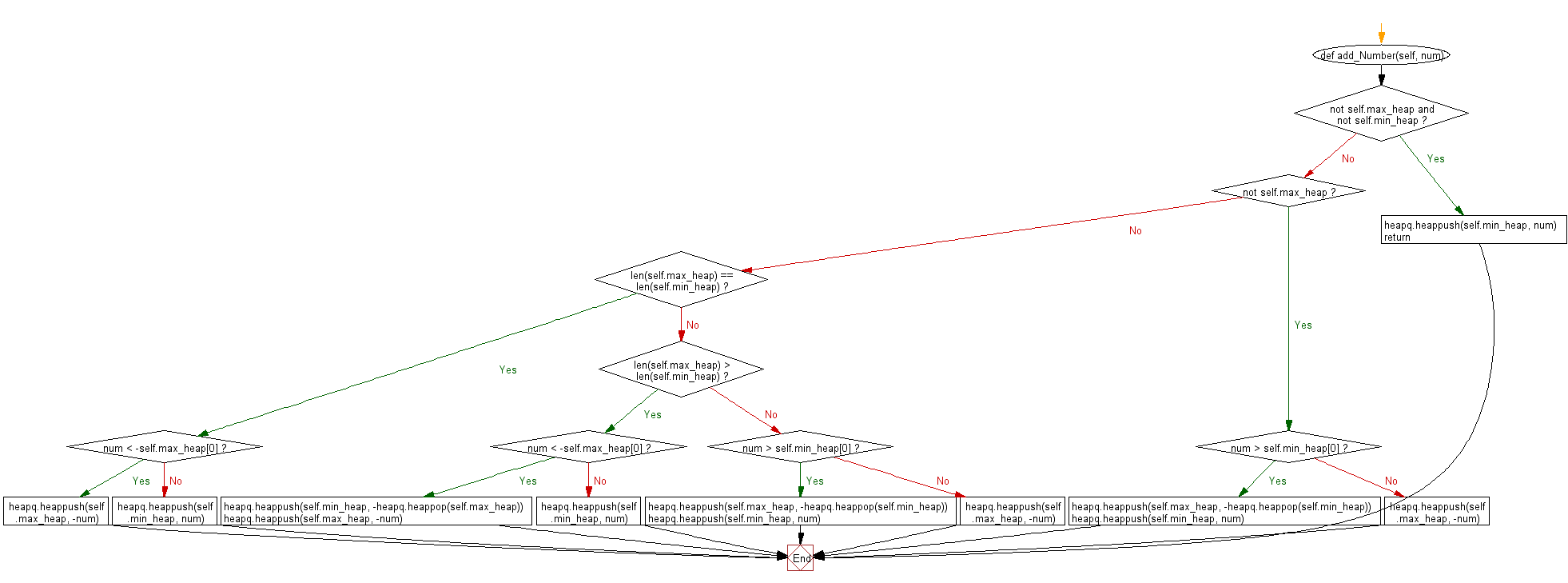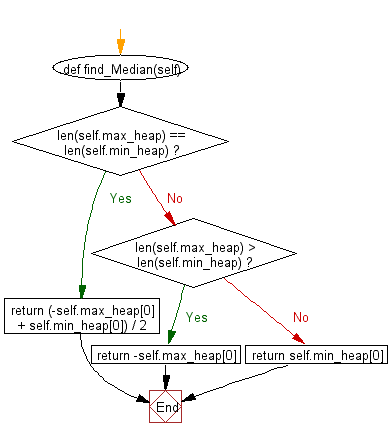﻿ Python heap queue algorithm: Compute the median of all elements - w3resource

# Python: Compute the median of all elements

## Python heap queue algorithm: Exercise-16 with Solution

Write a Python program that adds integer numbers from the data stream to a heapq and computes the median of all elements. Use the heap queue algorithm.

From Wikipedia "In statistics and probability theory, the median is the value separating the higher half from the lower half of a data sample, a population or a probability distribution. For a data set, it may be thought of as the "middle" value. For example, in the data set [1, 3, 3, 6, 7, 8, 9], the median is 6, the fourth largest, and also the fourth smallest, number in the sample. For a continuous probability distribution, the median is the value such that a number is equally likely to fall above or below it."

Sample Solution:

Python Code:

``````import heapq
class Median_Finder:

def __init__(self):
#initialize data structure
self.max_heap = []
self.min_heap = []

#type num: int, rtype: void
if not self.max_heap and not self.min_heap:
heapq.heappush(self.min_heap, num)
return
if not self.max_heap:
if num > self.min_heap:
heapq.heappush(self.max_heap, -heapq.heappop(self.min_heap))
heapq.heappush(self.min_heap, num)
else:
heapq.heappush(self.max_heap, -num)
return
if len(self.max_heap) == len(self.min_heap):
if num < -self.max_heap:
heapq.heappush(self.max_heap, -num)
else:
heapq.heappush(self.min_heap, num)
elif len(self.max_heap) > len(self.min_heap):
if num < -self.max_heap:
heapq.heappush(self.min_heap, -heapq.heappop(self.max_heap))
heapq.heappush(self.max_heap, -num)
else:
heapq.heappush(self.min_heap, num)
else:
if num > self.min_heap:
heapq.heappush(self.max_heap, -heapq.heappop(self.min_heap))
heapq.heappush(self.min_heap, num)
else:
heapq.heappush(self.max_heap, -num)

def find_Median(self):
#rtype: float
if len(self.max_heap) == len(self.min_heap):
return (-self.max_heap + self.min_heap) / 2
elif len(self.max_heap) > len(self.min_heap):
return -self.max_heap
else:
return self.min_heap
S = Median_Finder()
result = S.find_Median()
print(result)
result = S.find_Median()
print(result)
result = S.find_Median()
print(result)
```
```

Sample Output:

```1.5
2
3
```

Flowchart:Python Code Editor:

Have another way to solve this solution? Contribute your code (and comments) through Disqus.

What is the difficulty level of this exercise?

Test your Programming skills with w3resource's quiz.

﻿